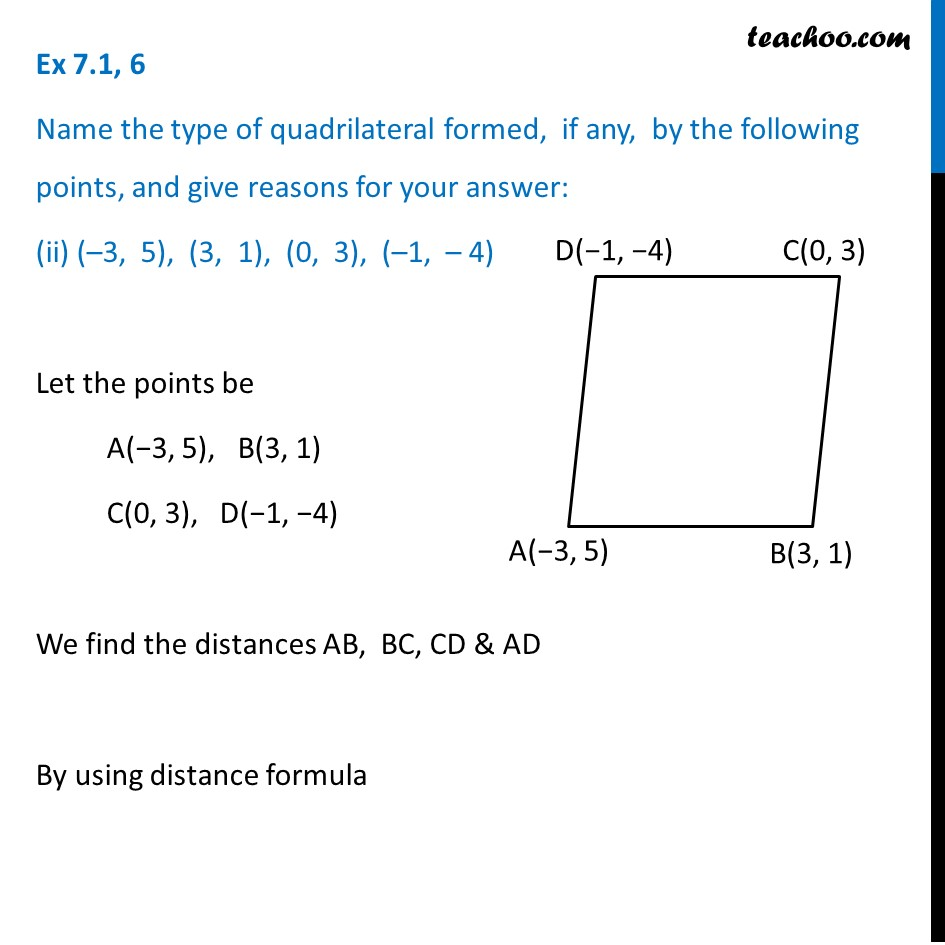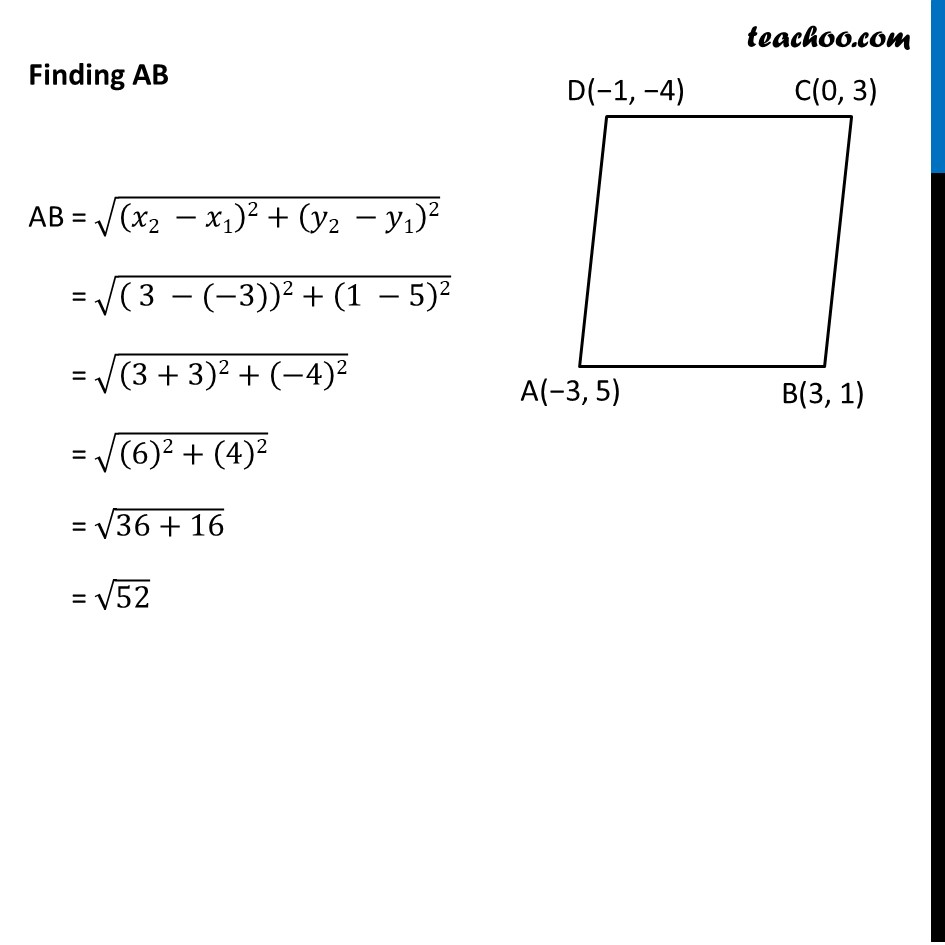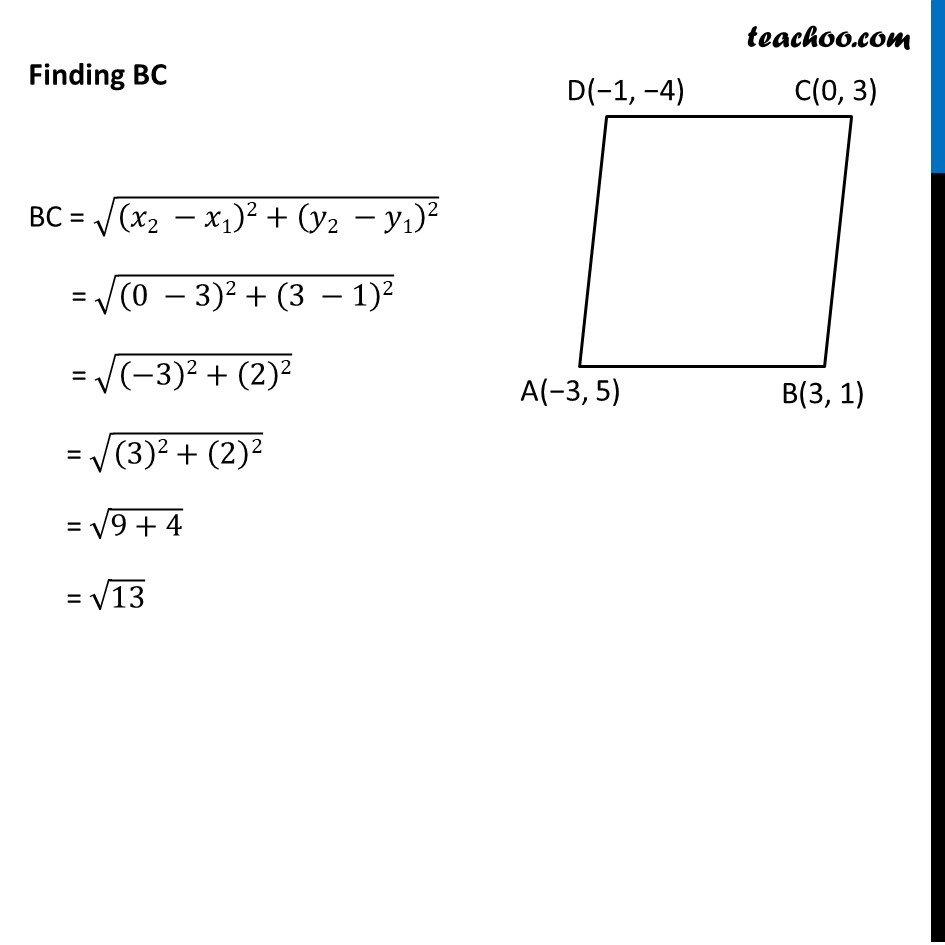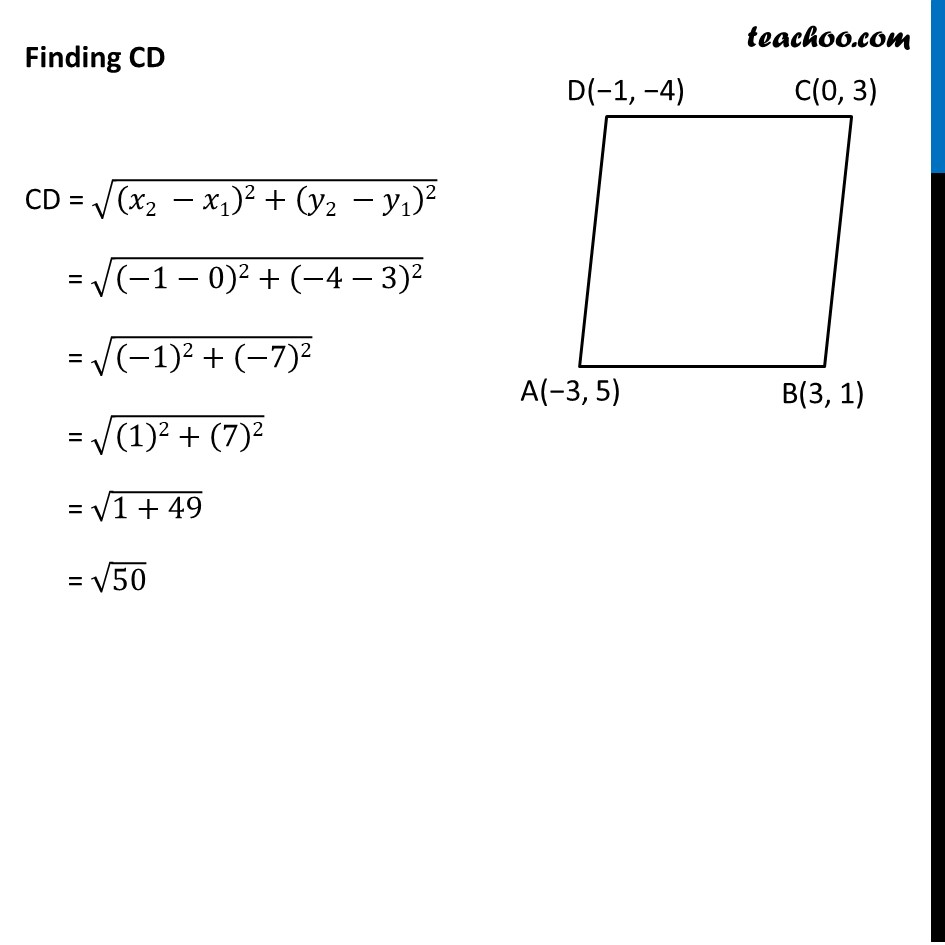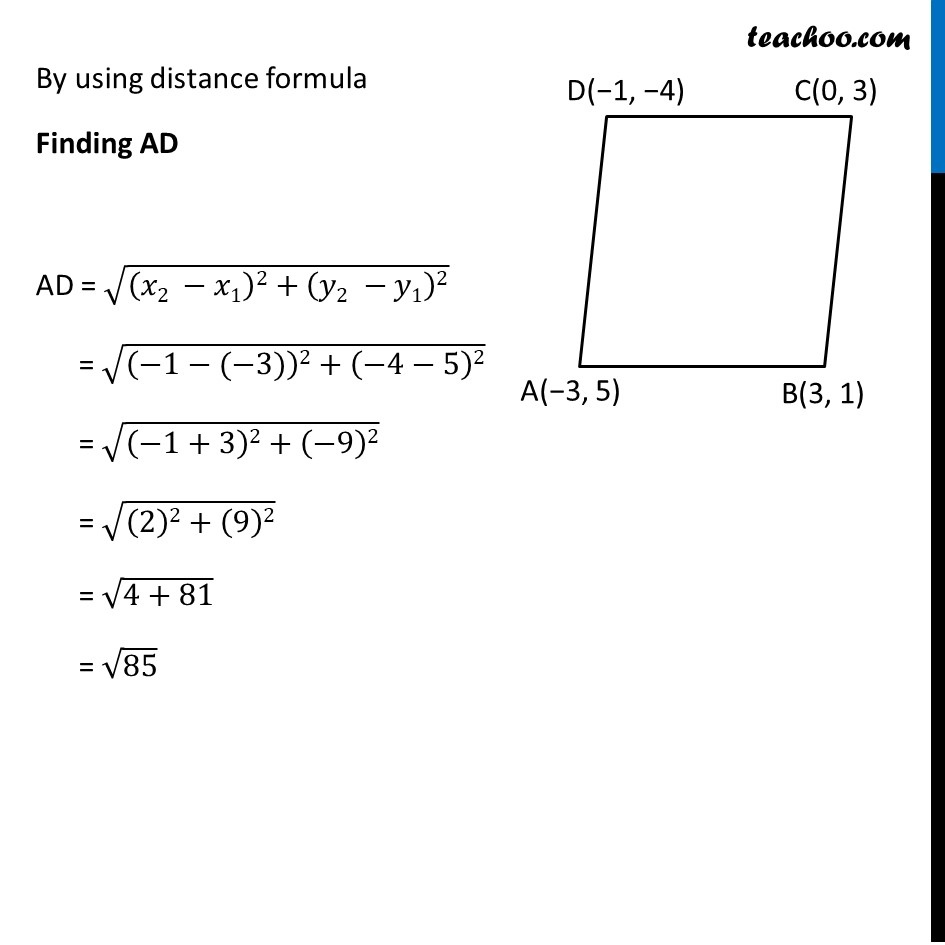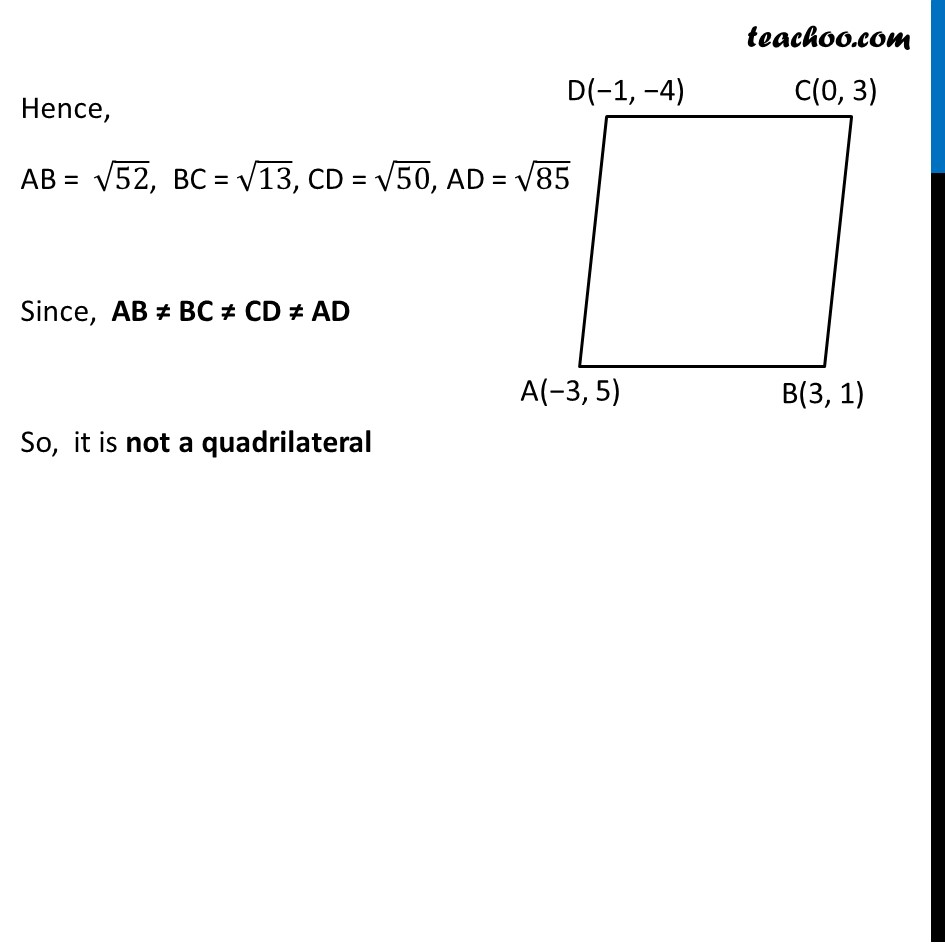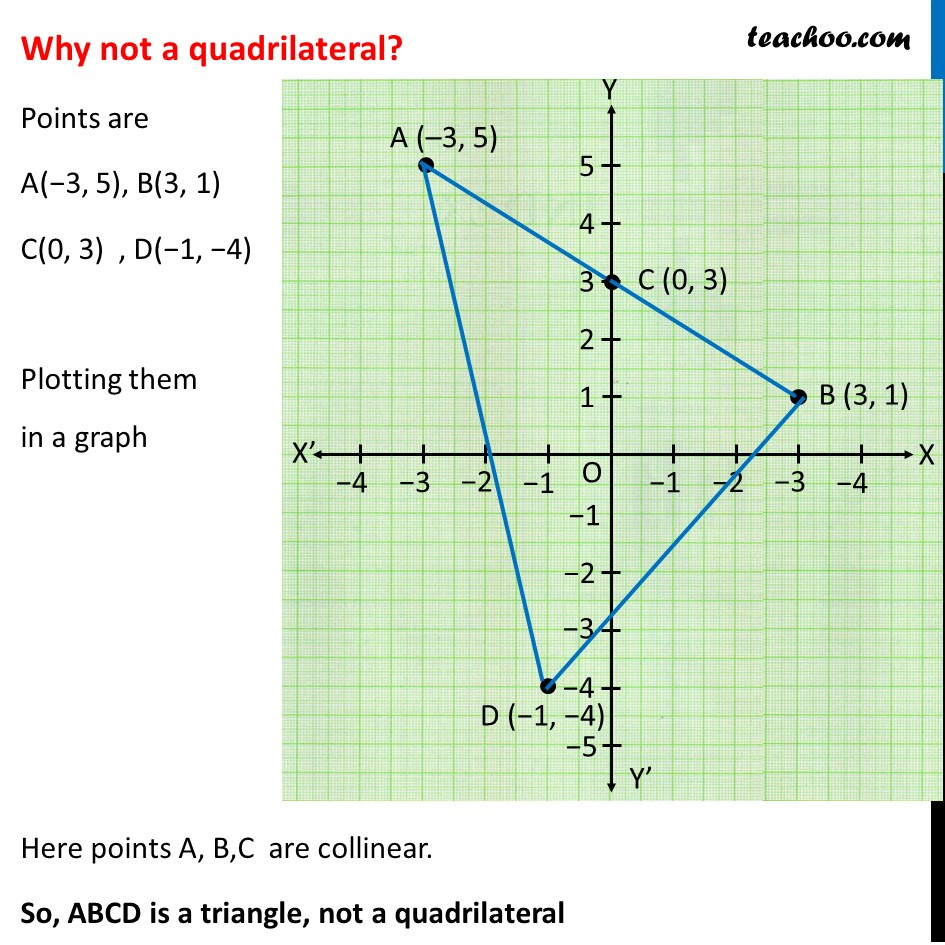1. Chapter 7 Class 10 Coordinate Geometry (Term 1)
2. Serial order wise
3. Ex 7.1

Transcript

Ex 7.1, 6 Name the type of quadrilateral formed, if any, by the following points, and give reasons for your answer: (ii) (–3, 5), (3, 1), (0, 3), (–1, – 4) Let the points be A(−3, 5), B(3, 1) C(0, 3), D(−1, −4) We find the distances AB, BC, CD & AD By using distance formula Finding AB AB = √((𝑥2 −𝑥1)2+(𝑦2 −𝑦1)2) = √(( 3 −(−3))2+(1 −5)2) = √((3+3)2+(−4)2) = √((6)2+(4)2) = √(36+16) = √52 Finding BC BC = √((𝑥2 −𝑥1)2+(𝑦2 −𝑦1)2) = √((0 −3)2+(3 −1)2) = √((−3)2+(2)2) = √((3)2+(2)2) = √(9+4) = √13 Finding CD CD = √((𝑥2 −𝑥1)2+(𝑦2 −𝑦1)2) = √((−1−0)2+(−4−3)2) = √((−1)2+(−7)2) = √((1)2+(7)2) = √(1+49) = √50 By using distance formula Finding AD AD = √((𝑥2 −𝑥1)2+(𝑦2 −𝑦1)2) = √((−1−(−3))2+(−4−5)2) = √((−1+3)2+(−9)2) = √((2)2+(9)2) = √(4+81) = √85 Hence, AB = √52, BC = √13, CD = √50, AD = √85 Since, AB ≠ BC ≠ CD ≠ AD So, it is not a quadrilateral Why not a quadrilateral? Points are A(−3, 5), B(3, 1) C(0, 3) , D(−1, −4) Plotting them in a graph Here points A, B,C are collinear. So, ABCD is a triangle, not a quadrilateral